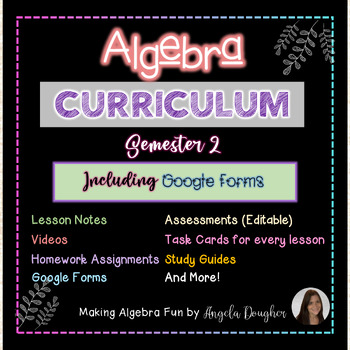# Algebra 1 curriculum BUNDLE (SEMESTER 2)Subject
Resource Type
Product Rating
File Type

Compressed Zip File

Be sure that you have an application to open this file type before downloading and/or purchasing.

150 MB|1500+
Share
9 Products in this Bundle
9 products
Bundle Description

These materials are valued at over \$350. Purchase as a bundle and save big!! This is the second half of my curriculum for Algebra 1 and it will continue to GROW. It includes 6 units of individual lessons with full examples shown along with practice problems, homework, study guides, and 2 editable quizzes and tests for each unit. There is a review and final exam covering all 6 units as well. Each unit includes games related to the unit material.

All lessons are 100% prep free and with answer keys make it easy for students who were absent to catch up. Click each unit for details of what's included in that unit. You can also check out the games by clicking on the links.

General Interactive Game to be used with any lesson:

Scratch off ticket INCENTIVE for good behavior and success ANY TOPIC

10 Algebra SELF CHECK worksheets EXPRESSIONS EQUATIONS INEQUALITIES

Included Units:

Unit 7 Lessons:

Lesson 1 Classifying Polynomials and Addition/Subtraction of Polynomials

Lesson 2 Multiplying Polynomials

Lesson 3 Dividing Polynomials

Lesson 4 Multi-Step and Applications with Polynomials

Study Guides & 2 Editable Quizzes and Tests

Algebra Factoring Polynomials BUNDLE Lesson Notes Practice Homework Quiz & Test

Unit 8 Lessons:

Lesson 1 Greatest Common Factor

Lesson 2 x2 + bx + c

Lesson 3 ax2 + bx + c

Lesson 4 Difference of two perfect squares and perfect square trinomials

Lesson 5 Grouping

Lesson 6 Factoring Completely

Study Guides & 2 Editable Quizzes and Tests

Algebra Quadratic Equations BUNDLE Lesson Notes Practice Homework Quiz & Test

Unit 9 Lessons:

Lesson 1 Introduction to Quadratic Equations

Lesson 2 Graphing Quadratic Equations

Lesson 3 Quadratic Equations in Vertex Form

Lesson 4 Solving Quadratic Equations by Graphing

Lesson 5 Solving Quadratic Equations by Factoring

Lesson 6 Solving Quadratic Equations by Finding Square Roots

Lesson 7 Solving Quadratic Equations by Quadratic Formula

Study Guides & 2 Editable Quizzes and Tests

Unit 10 Lessons:

Lesson 1 Simplifying Rational Expressions

Lesson 2 Multiplying Rational Expressions

Lesson 3 Dividing Rational Expressions

Lesson 4 Adding & Subtracting Rational Expressions with Like Bases

Lesson 5 Adding & Subtracting Rational Expressions with Unlike Bases

Lesson 6 Applications

Lesson 7 Solving Rational Equations

Lesson 8 Solving Rational Equations with Extraneous Solutions

Study Guides & 2 Editable Quizzes and Tests

Algebra Radical Expressions & Equation Lesson Notes Homework Quiz & Test

Unit 11 Lessons:

Lesson 1 Introduction to Radicals

Lesson 2 Cube Roots

Lesson 3 Simplifying Square Roots with Variables

Lesson 4 Simplifying Cube Roots with Variables

Lesson 6 Multiplying Radicals

Lesson 7 Dividing Radicals Part 1

Lesson 8 Dividing Radicals Part 2

Lesson 9 Solving Radical Equations

Lesson 10 Applications

Study Guides & 2 Editable Quizzes and Tests

Algebra Probability & Statistics 10 Lessons Notes Homework Quiz & Test BUNDLE

Unit 12 Lessons:

Lesson 1 Simple Probability

Lesson 2 Theoretical vs. Experimental Probability

Lesson 3 Permutations

Lesson 4 Combinations

Lesson 5 Probability using Permutations & Combinations

Lesson 6 Compound Events

Lesson 7 Surveys & Samples

Lesson 8 Measures of Central Tendency

Lesson 9 Stem & Leaf Plots, Histograms & Box & Whisker Plots

Lesson 10 Scatter Plots & Lines of Best Fit

Study Guides & 2 Editable Quizzes and Tests

A final exam and study guide covering units 1-12 are included. This exam is given in 3 formats. 1. Multiple choice (editable) 2. Short Answer (editable) 3. Mix of Multiple choice and short answer

These materials are valued at over \$350. Purchase as a bundle and save big!!

As items are added the price will increase. Purchase now for the best deal and take advantage of all future additions free of charge!

For the full course check out my Algebra 1 curriculum BUNDLE with growing GAME PACK.

Interested in the curriculum WITHOUT the game pack?

Algebra 1 curriculum BUNDLE Lesson Notes Homework Study Guides Quizzes & Tests

Interested in games only to add to your curriculum?

Algebra Game Pack 50 Interactive Games on a variety of Algebra topics

The purchase of this product entitles you to personal use within one classroom. Sharing among colleagues is not permitted. Posting online or selling is strictly prohibited. Thank you for respecting my time and work.

Total Pages
1500+
Included
Teaching Duration
N/A
Report this Resource
Reported resources will be reviewed by our team. Report this resource to let us know if this resource violates TpT’s content guidelines.